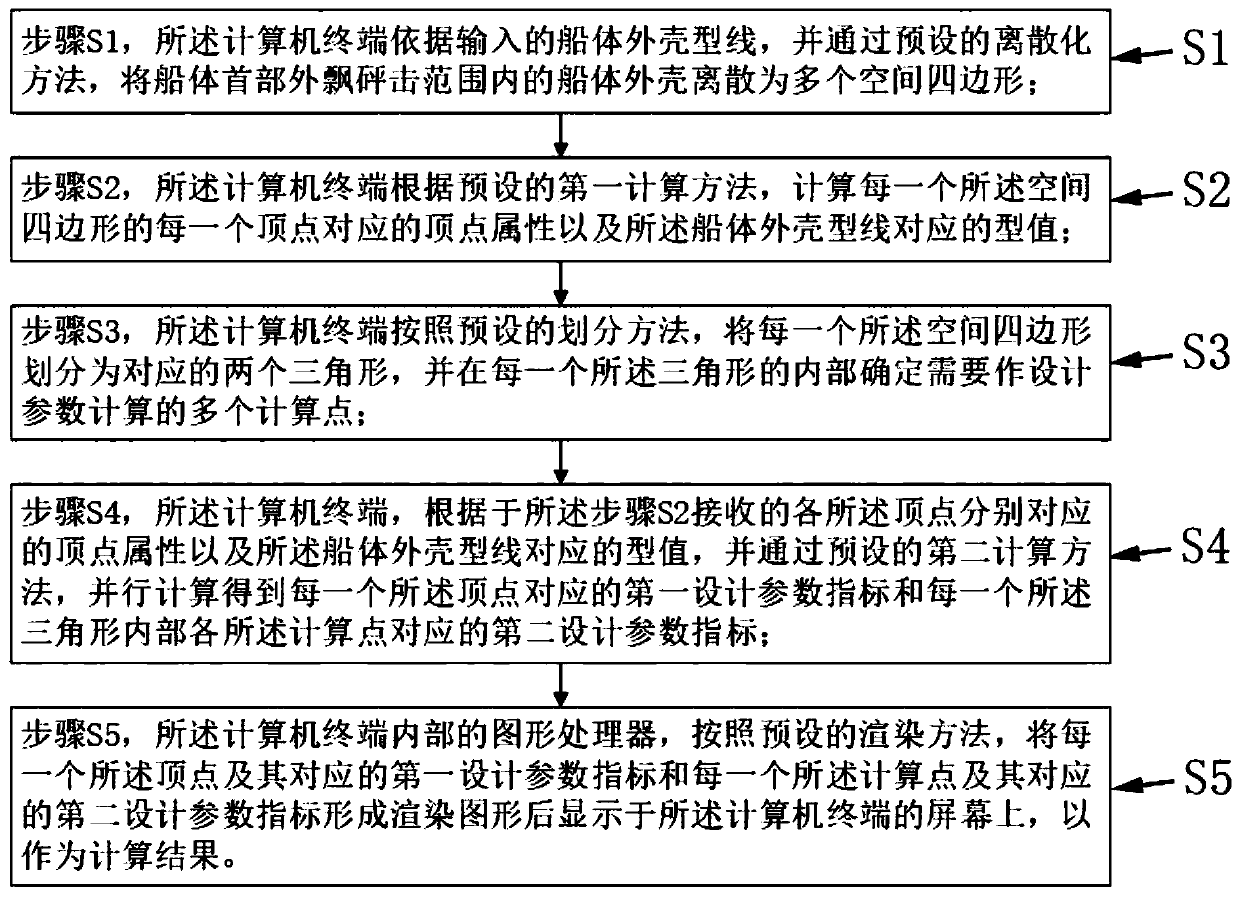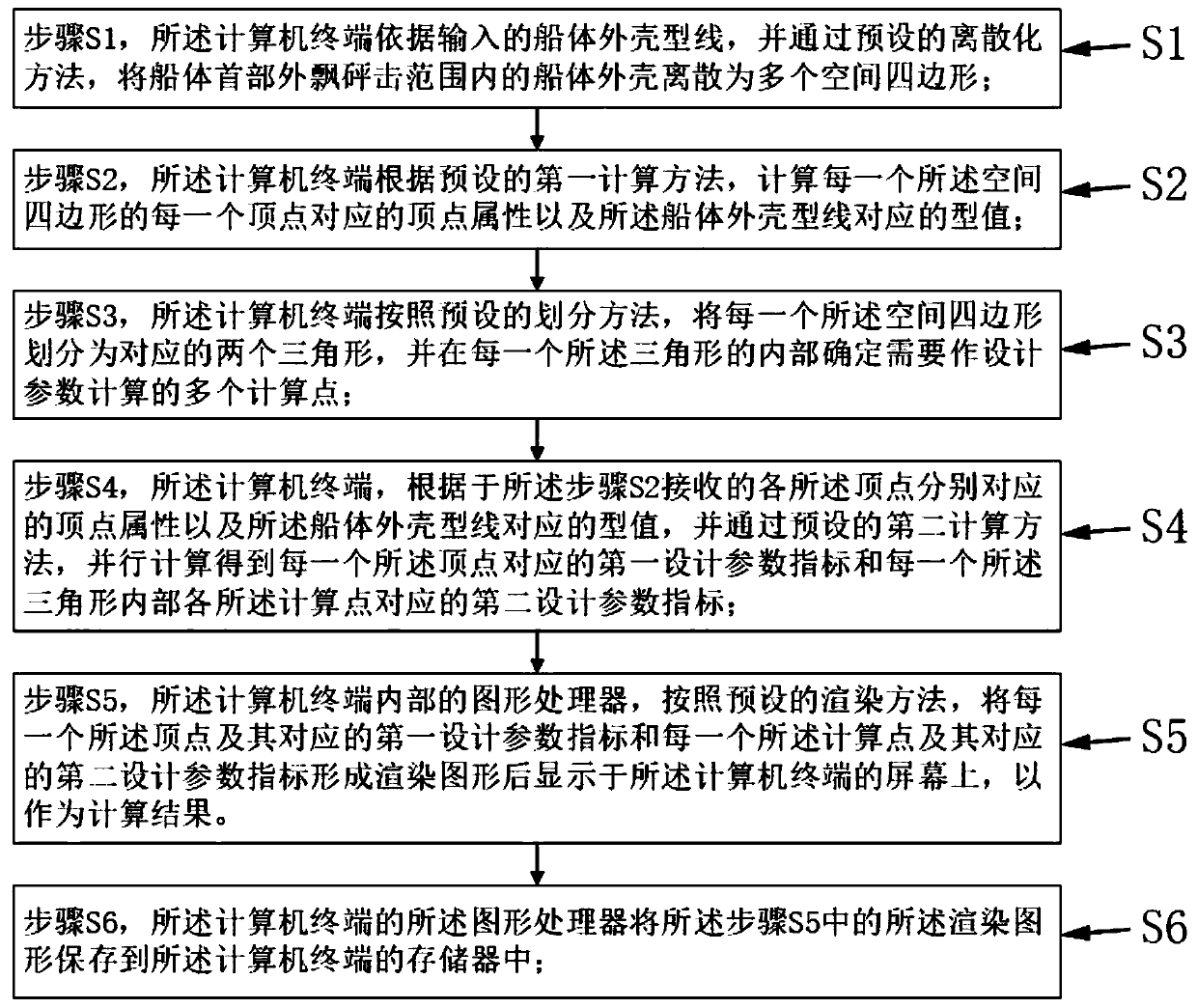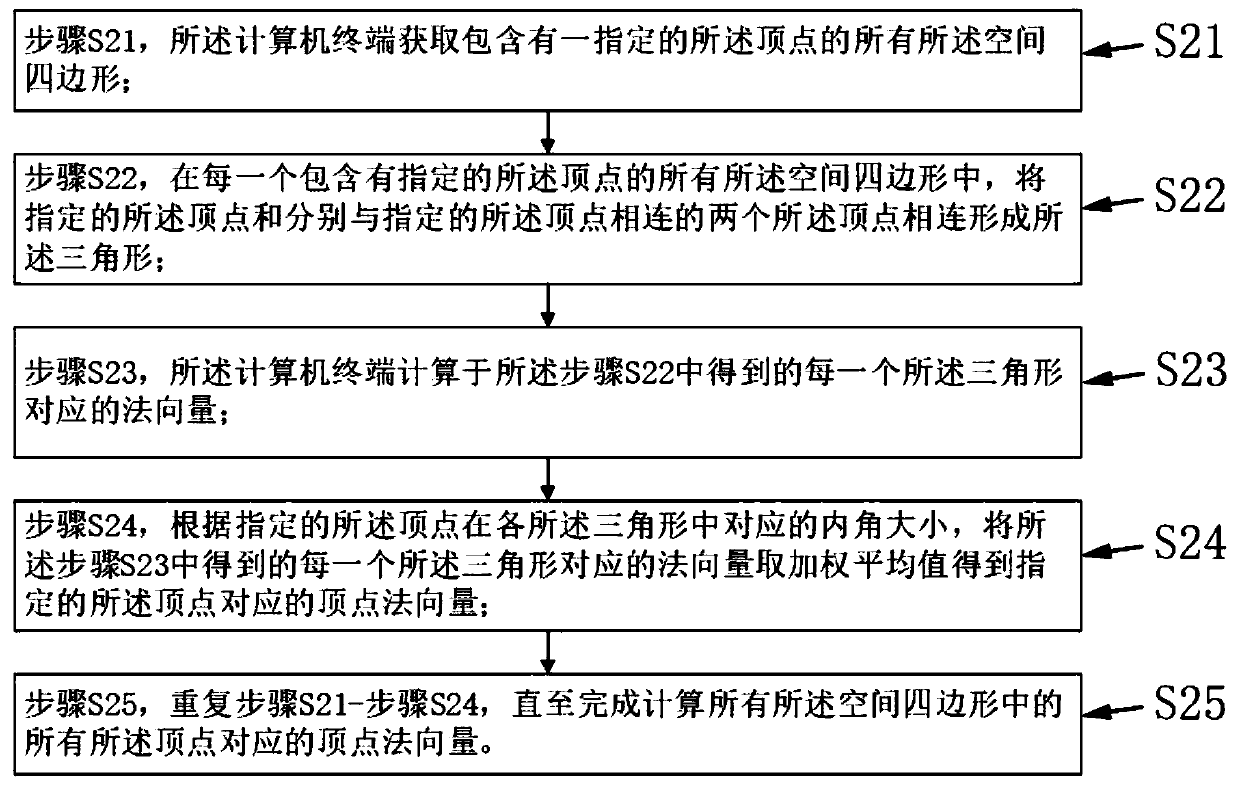# A hull head drift flare-out slamming parallel computing method based on a three-dimensional model

## A parallel computing and three-dimensional model technology, applied in complex mathematical operations, etc., can solve problems such as long calculation time, complex calculation principles, and reduced work efficiency, and achieve fast calculation speed, simple calculation principle, and intuitive and clear results.

Pending Publication Date: 2019-05-07
708TH RES INST OF CSSC
11 Cites 2 Cited by

## AI-Extracted Technical Summary

### Problems solved by technology

The traditional calculation of various parameters of the hull design by manually measuring data greatly reduces work efficiency, and the data measured by the ship designer cannot intuitively represent the three-dimensional structure of the hull, such as the hull shell. The norm...
View more

## Abstract

The invention discloses a hull head flare-out slamming parallel computing method based on a three-dimensional model. The hull head flare-out slamming parallel computing method comprises the followingsteps that S1, dispersing a hull shell within the hull head outwards-floating slamming range into a plurality of spatial quadrangles through a computer terminal; S2, calculating the vertex attribute corresponding to each vertex of each spatial quadrangle; S3, dividing each spatial quadrangle into two corresponding triangles, and determining a plurality of calculation points needing parameter calculation in each triangle; S4, calculating a first design parameter index corresponding to each vertex and a second design parameter index corresponding to each calculation point based on each vertex attribute; And S5, according to a preset rendering method, displaying each vertex, each calculation point and the corresponding first design parameter index and the corresponding second design parameterindex on a computer terminal screen, the principle is simple, the calculation speed is high, and various design parameters of the ship can be visually displayed.

Application Domain

Complex mathematical operations

Technology Topic

Three dimensional modelFlare +4

## Image

•••## Examples

• Experimental program(2)

### Example Embodiment

 Example one:
 Please refer to figure 1 , The first embodiment of the present invention provides a parallel calculation method for the slamming of the bow of a ship based on a three-dimensional model, which runs on a computer terminal and specifically includes the following steps:
 Step S1: The computer terminal discretizes the hull hull within the slamming range of the hull bow into multiple spatial quadrilaterals according to the input hull hull profile and through a preset discretization method;
 A plurality of the space quadrilaterals and the hull hull profile line constitutes a hull bow slamming calculation database; the hull hull profile includes a water line and a cross section line, and the hull hull profile line can be processed by existing graphics Software processing formation.
 Step S2: The computer terminal calculates the vertex attribute corresponding to each vertex of each spatial quadrilateral and the shape value corresponding to the hull hull profile according to the preset first calculation method;
 The vertex attribute of each vertex includes the coordinate value of each vertex and the corresponding vertex normal vector;
 The profile value of the hull hull profile is the coordinate value of each point that determines the spatial position of the profile.
 Step S3: The computer terminal divides each of the spatial quadrilaterals into two corresponding triangles according to a preset dividing method, and determines multiple calculation points that need to be calculated for design parameters in each of the triangles;
 Step S4, the computer terminal, according to the vertex attribute corresponding to each of the vertices received in the step S2 and the property corresponding to the hull hull profile, and using a preset second calculation method to obtain each A first design parameter index corresponding to one of the vertices and a second design parameter index corresponding to each of the calculation points inside each of the triangles;
 The first design parameter index is one of the local waterline angle of each of the vertices, the local impact angle of the bow on each of the vertices, and the local linear angle of the tangent from the horizontal line to each of the vertices. Kind or more
 The second design parameter index is the local waterline angle of each of the calculation points inside the triangle, the local impact angle of the bow at each calculation point, and the connection from the horizontal line to each calculation point. One or more of the local linear angles of the tangent.
 The computer terminal is based on the first design parameter index and the second design parameter index, and according to the preset second calculation method to calculate the bow impact design pressure, the net thickness of the side shell plate, the frame and the Design parameters such as the effective net shaping section modulus of the effective plate, the net thickness of the frame web, the net section modulus of the hull support member, and the net shear area of ​​the hull support member web;
 Step S5, the graphics processor inside the computer terminal, according to a preset rendering method, converts each of the vertex and its corresponding first design parameter index and each of the calculation points and its corresponding second design parameter The indicator forms a rendered graphic and is displayed on the screen of the computer terminal as a calculation result.
 Specifically, the preset rendering method is that the shader in the graphics processor is based on the different vertex attributes corresponding to each of the vertices, the different calculation point attributes corresponding to each of the calculation points, and the hull hull. The type value of the type line, using different colors and color depths to distinguish each of the vertices of each of the spatial quadrilaterals and the calculation points in the triangles, and finally display the graphics on the screen of the computer terminal after rendering .
 In a preferred solution of the first embodiment of the present invention, please refer to image 3 In the step S2, the method for the computer terminal to calculate the vertex normal vector corresponding to each vertex of each of the spatial quadrilaterals according to the preset first calculation method specifically includes the following steps:
 Step S21, the computer terminal obtains all the spatial quadrilaterals that include a designated vertex;
 Step S22, in each of all the spatial quadrilaterals containing the designated vertex, connect the designated vertex and the two vertices respectively connected to the designated vertex to form the triangle;
 Step S23, the computer terminal calculates the normal vector corresponding to each of the triangles obtained in the step S22;
 Step S24: Take a weighted average of the normal vectors corresponding to each of the triangles obtained in step S23 to obtain the vertex corresponding to the designated vertex according to the size of the inner angle corresponding to the designated vertex in each of the triangles Normal vector
 Step S25, repeating steps S21 to S24 until the vertex normal vectors corresponding to all the vertices in all the space quadrilaterals are calculated.
 Please refer to Figure 4 , The discretization method described in step S1 specifically includes the following steps:
 Step A1: Divide each of the waterlines up and down on the hull shell into equal number of line segments in a bisecting manner, and then record the bisecting nodes on each of the waterlines in turn along the bow to stern direction; Record the first node, the second node, the third node... until the final nth node is recorded on each of the water lines;
 Step A2: Connect the bisecting nodes in the same order on each of the water lines in order in the height direction to form a plurality of isoparametric lines; each of the water lines and the isoparametric lines are mutually divided to form a plurality of the spatial quadrilaterals . For example, the first node of each waterline is connected up and down to form a first isoparm that divides each waterline; and then connected in the same way to form a second isoparm, a third, etc. that divide each waterline Parametric lines...until the formation of the nth isoparm; each of the water lines and each of the isoparms is divided from each other to form a plurality of the space quadrilaterals.
 In order to ensure calculation accuracy, the grid size corresponding to each of the space quadrilaterals is preferably less than or equal to 200mm*200m.
 In a preferred solution of the first embodiment of the present invention, in the step S4, the second calculation method includes a barycentric coordinate interpolation method, and the barycentric coordinate interpolation method is calculated by the following formula to obtain each position in each triangle The second design parameter index corresponding to the calculation point:
 p=λ 1 a+λ 2 b+λ 3 c
 among them,
 P is used to represent the second design parameter index,
 a, b, and c are respectively the first design parameter index of the three vertices A, B, and C corresponding to the triangle;
 The ratio of the area of ​​triangle PBC, triangle PCA and triangle PAB is λ 1 : Λ 2 : Λ 3 , And λ 1 +λ 2 +λ 3 =1;
 P is used to represent the calculation point inside the triangle.
 In a preferred solution of Embodiment 1 of the present invention, the computer terminal calculates the impact of each of the vertices of each of the spatial quadrilaterals and the calculated points of each of the triangles by the following formula: design pressure:

 among them,
 P FB Used to indicate the impact design pressure of the bow of the hull;
 f FB It is used to represent the longitudinal distribution coefficient of the impact pressure on the bow of the hull at each of the vertices or the calculation points;
 V im Used to represent the impact velocity of each of the vertices or each of the calculation points;
 γ wl Used to represent the local impact angle of the bow of each of the vertices or each of the calculation points;
 C FB It is used to indicate the vertical position coefficient of each vertex or each calculation point.
 In a preferred solution of the first embodiment of the present invention, in the step S4, the parallel calculation process is performed in the graphics processor inside the computer terminal, and then the parallel result is directly rendered in the step S5 And displayed on the screen of the computer terminal.
 In addition, it should be noted that the coordinate value of each vertex in the vertex attribute corresponding to each vertex and the shape of the hull hull profile are calculated by using the first calculation method in the step S2. The value method is a calculation method in the prior art; in the step S4, the second calculation method is used to calculate the first design parameter index corresponding to each vertex and each of the triangles The method for calculating the second design parameter index corresponding to the point is also the calculation method in the prior art, and will not be elaborated here.

### Example Embodiment

 Embodiment two:
 The difference between the second embodiment and the first embodiment is that, please refer to figure 2 , The parallel calculation method for the slamming outside the bow of a ship based on the three-dimensional model provided in the second embodiment of the present invention further includes:
 Step S6, the graphics processor of the computer terminal saves the rendered graphics in the step S5 to the memory of the computer terminal;
 The computer terminal also includes a user interaction module, the user interaction module is connected to the memory, and is provided for the user to select any point in the rendered graphics on the screen of the computer terminal to obtain it from the memory The first design parameter index corresponding to the selected arbitrary point or the vertex closest to the selected arbitrary point or the second design parameter index corresponding to the calculated point stored in And displayed on the screen of the computer terminal.
 Specifically, the user can click any point position of the three-dimensional model of the ship hull displayed on the screen of the computer terminal by clicking or touching with a mouse, and the central processing unit of the computer terminal sends the click signal input by the user For the user interaction module, after receiving the click signal, the user interaction module retrieves all points corresponding to the arbitrary point or the vertex closest to the arbitrary point in the storage unit. The first design parameter index or the second design parameter index corresponding to the calculation point is displayed on the screen of the computer terminal, and preferably displayed near the arbitrary point position.
 In summary, through the three-dimensional model-based parallel calculation method for slamming hull bows provided by the present invention, the hull structure designer does not need to repeatedly manually measure the relevant data of the hull, calculate the angles of all hull bow slamming areas and other The calculation of the design parameter index is automatically completed on the computer terminal; the calculation principle of the present invention is simple, the calculation speed is faster, and the calculation result can be displayed on the screen of the computer terminal intuitively. The designer only needs to click on the relevant position of the hull displayed on the screen, The design parameter indicators corresponding to the hull position can be displayed, and the calculation results are intuitive and clear.

## PUM## Description & Claims & Application Information

We can also present the details of the Description, Claims and Application information to help users get a comprehensive understanding of the technical details of the patent, such as background art, summary of invention, brief description of drawings, description of embodiments, and other original content. On the other hand, users can also determine the specific scope of protection of the technology through the list of claims; as well as understand the changes in the life cycle of the technology with the presentation of the patent timeline. Login to view more.

## Method, device and system for detecting bridge tap

Owner:HUAWEI TECH CO LTD

## Intelligent layer-burning boiler extinguishing boundary detecting system

ActiveCN104864411AThe calculation principle is simplesmall amount of calculation
Owner:XIAMEN OPTIKOM AUTOMATIC CONTROL TECH CO LTD

## Determination method and device for critical clearing time

Owner:STATE GRID CORP OF CHINA +1

## Defect area calculation method and system for pipeline detection

ActiveCN112595266AThe calculation principle is simpleThe calculation result is accurate
Owner:WUHAN EASY SIGHT TECH

## Active infrared action identifying method

Owner:PIZHOU GUOLONG ELECTRIC

## Point clouds simplifying system and method

InactiveCN104794687Asimple methodcalculation speed
Owner:HONG FU JIN PRECISION IND (SHENZHEN) CO LTD +1

## Tunnel back break numerical calculation method based on laser point cloud measurement

PendingCN114511678AThe calculation principle is simpleWide range of applications

## Template image global searching method based on mask matrix and fast Fourier transform

ActiveCN106056158Aovercoming complexitycalculation speed
Owner:XIDIAN UNIV

## Classification and recommendation of technical efficacy words

• calculation speed
• The calculation principle is simple

## Network flow rate abnormality detecting method based on super stochastic theory

InactiveCN101286897Acalculation speed
Owner:HUAZHONG UNIV OF SCI & TECH

## Parallel connection equipment opening type motion control card based on two-stage DSP and control method thereof

InactiveCN1808319Acalculation speedReduce data communication pressure
Owner:TIANJIN UNIV

## Method for realizing server hardware acceleration by using FPGA (field programmable gate array)

InactiveCN104657308Acalculation speedIncrease bus bandwidth
Owner:LANGCHAO ELECTRONIC INFORMATION IND CO LTD

## Navigation image matching filtering method based on K-means clustering algorithm

Owner:GUANGDONG BONA ROBOT CORP LTD

## Method for ordering microblog network node weights based on multi-link

InactiveCN102214212Asmall scalecalculation speed
Owner:NORTHWESTERN POLYTECHNICAL UNIV
Who we serve
• R&D Engineer
• R&D Manager
• IP Professional
Why Eureka